# For each of the following solute-solvent combinations, state the sign and relative magnitudes for ∆ H 1 , ∆ H 2 , ∆ H 3 , and ∆ H soln , (as defined in Fig. 10-1 of the text). Explain your answers. a. and b. and c. and d. and### Chemistry: An Atoms First Approach

2nd Edition
Steven S. Zumdahl + 1 other
Publisher: Cengage Learning
ISBN: 9781305079243

#### Solutions

Chapter
Section### Chemistry: An Atoms First Approach

2nd Edition
Steven S. Zumdahl + 1 other
Publisher: Cengage Learning
ISBN: 9781305079243
Chapter 10, Problem 98AE
Textbook Problem
241 views

## For each of the following solute-solvent combinations, state the sign and relative magnitudes for ∆H1, ∆H2, ∆H3, and ∆Hsoln, (as defined in Fig. 10-1 of the text). Explain your answers.a.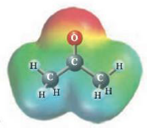and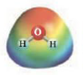b.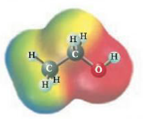and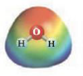c.and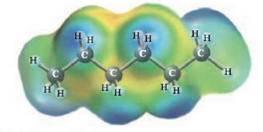d.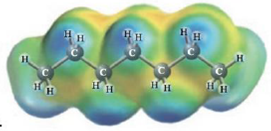and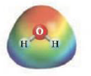a)

Interpretation Introduction

Interpretation: The dissolution of the given following solute and solvent has to be explained.

Concept Introduction: Concept introduction:

Raoult's law:

The mole fraction of a solute is related to the vapor pressure of the solution thus,

Psolution=P°solventXsolvent......(1)Pisvapor pressureof the solutionsolventpressureof the solventXsolvent mole fraction ofsolvent

### Explanation of Solution

To find the Acetone and water polarity

CH3COCH3 and H2O

The electrostatic possible drawing illustrates that acetone ( CH3COCH3 ), like water, is a polar substance (each has a red end indicating the partial negative end of the dipole moment and a blue end indicating the partial positive end). For a polar solute in a polar solvent, ΔH1 and ΔH2 will be large and positive, whilst ΔH3 will be a large negative value

b)

Interpretation Introduction

Interpretation: The dissolution of the given following solute and solvent has to be explained.

Concept Introduction: Concept introduction:

Raoult's law:

The mole fraction of a solute is related to the vapor pressure of the solution thus,

Psolution=P°solventXsolvent......(1)Pisvapor pressureof the solutionsolventpressureof the solventXsolvent mole fraction ofsolvent

c)

Interpretation Introduction

Interpretation: The dissolution of the given following solute and solvent has to be explained.

Concept Introduction: Concept introduction:

Raoult's law:

The mole fraction of a solute is related to the vapor pressure of the solution thus,

Psolution=P°solventXsolvent......(1)Pisvapor pressureof the solutionsolventpressureof the solventXsolvent mole fraction ofsolvent

d)

Interpretation Introduction

Interpretation: The dissolution of the given following solute and solvent has to be explained.

Concept Introduction: Concept introduction:

Raoult's law:

The mole fraction of a solute is related to the vapor pressure of the solution thus,

Psolution=P°solventXsolvent......(1)Pisvapor pressureof the solutionsolventpressureof the solventXsolvent mole fraction ofsolvent

### Still sussing out bartleby?

Check out a sample textbook solution.

See a sample solution

#### The Solution to Your Study Problems

Bartleby provides explanations to thousands of textbook problems written by our experts, many with advanced degrees!

Get Started

Find more solutions based on key concepts
Which of these B vitamins is (are) present only in foods of animal origin? a. niacin b. vitamin c. riboflavin d...

Nutrition: Concepts and Controversies - Standalone book (MindTap Course List)

Where would you go on Earth to have a celestial pole at your zenith?

Horizons: Exploring the Universe (MindTap Course List)

A Show that the time constant RC has the SI units of seconds.

Physics for Scientists and Engineers: Foundations and Connections

Can a storm surge be predicted?

Oceanography: An Invitation To Marine Science, Loose-leaf Versin

A conductor consists of a circular loop of radius R = 15.0 cm and two long, straight sections as shown in Figur...

Physics for Scientists and Engineers, Technology Update (No access codes included)

Write the name of the compound whose carbon skeleton is given.

Introductory Chemistry: An Active Learning Approach